Question

# Consider a unity feedback control system with open loop transfer function KG(G) s(s+2)(s + 6) 1....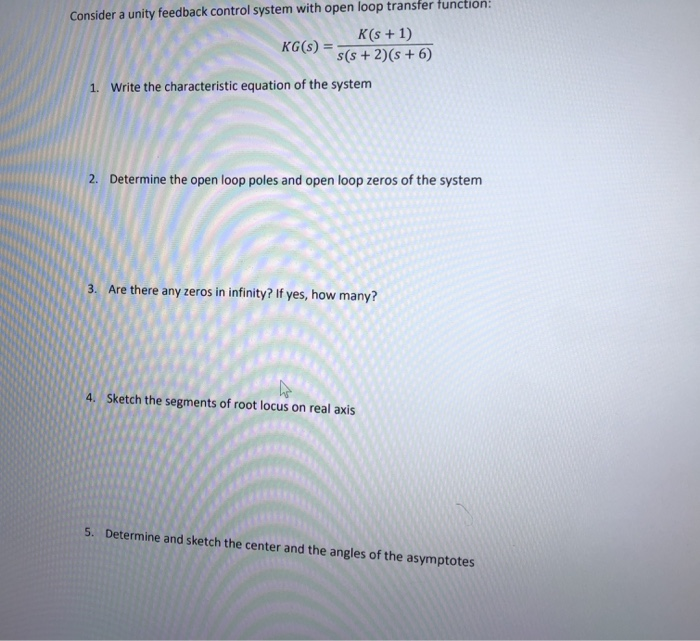Consider a unity feedback control system with open loop transfer function KG(G) s(s+2)(s + 6) 1. Write the characteristic equation of the system 2. Determine the open loop poles and open loop zeros of the system 3. Are there any zeros in infinity? If yes, how many? 4. Sketch the segments of root locus on real axis 5. Determine and sketch the center and the angles of the asymptotes

We need at least 10 more requests to produce the answer.

0 / 10 have requested this problem solution

The more requests, the faster the answer.

All students who have requested the answer will be notified once they are available.

#### Earn Coins

Coins can be redeemed for fabulous gifts.

Similar Homework Help Questions
• ### Problem 2 Consider a unity feedback system with open-loop transfer function: K(s+10) s(s+5) (s+6) (s+8) a)...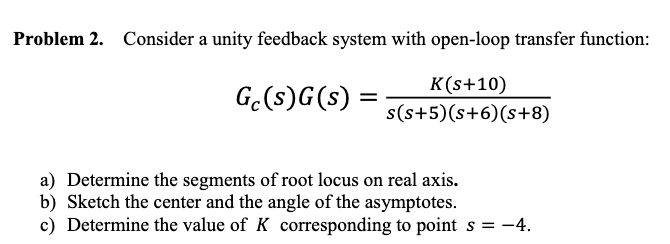Problem 2 Consider a unity feedback system with open-loop transfer function: K(s+10) s(s+5) (s+6) (s+8) a) Determine the segments of root locus on real axis. b) Sketch the center and the angle of the asymptotes c) Determine the value of K corresponding to point s =-4.

• ### Problem 2 For the unity feedback system below in Figure 2 G(s) Figure 2. With (8+2) G(s) = (a) Sk...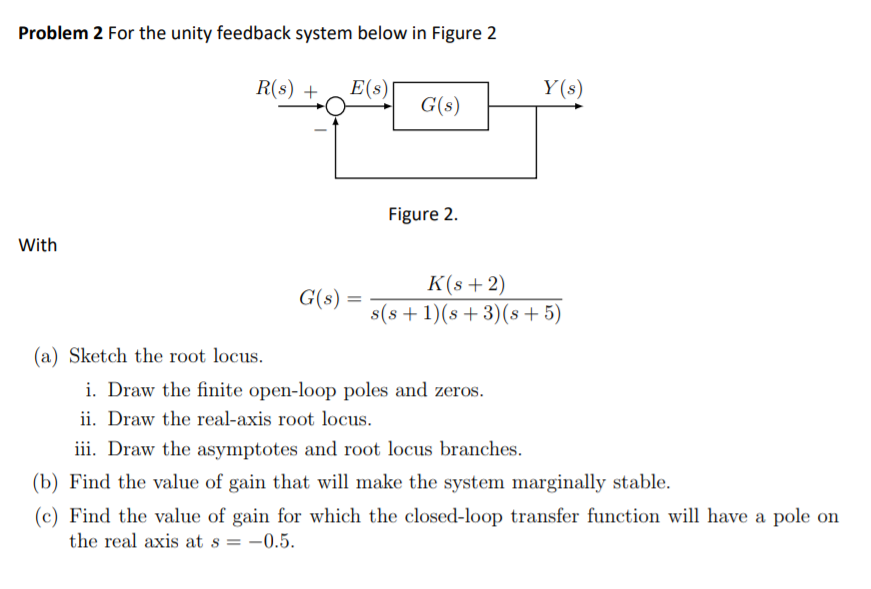Problem 2 For the unity feedback system below in Figure 2 G(s) Figure 2. With (8+2) G(s) = (a) Sketch the root locus. 1. Draw the finite open-loop poles and zeros. ii. Draw the real-axis root locus iii. Draw the asymptotes and root locus branches. (b) Find the value of gain that will make the system marginally stable. (c) Find the value of gain for which the closed-loop transfer function will have a pole on the real axis at s...

• ### Lectures 15-18: Root-locus method 5.1 Sketch the root locus for a unity feedback system with the ...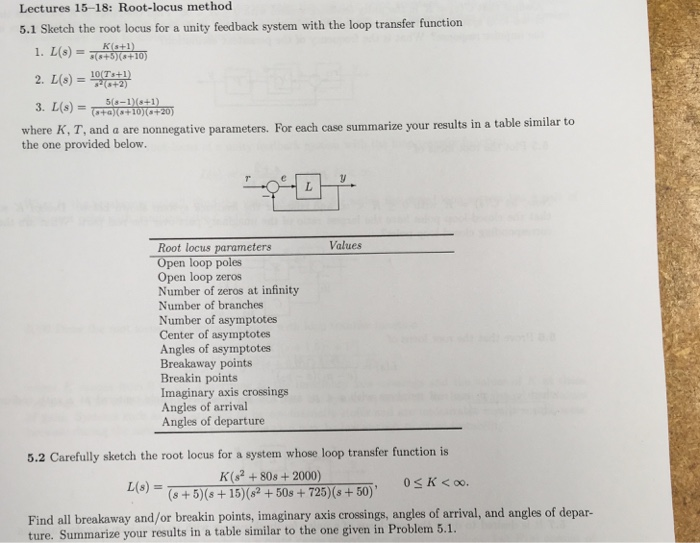help on #5.2 L(s) is loop transfer function 1+L(s) = 0 lecture notes: Lectures 15-18: Root-locus method 5.1 Sketch the root locus for a unity feedback system with the loop transfer function (8+5(+10) .2 +10+20 where K, T, and a are nonnegative parameters. For each case summarize your results in a table similar to the one provided below. Root locus parameters Open loop poles Open loop zeros Number of zeros at infinity Number of branches Number of asymptotes Center of...

• ### 2. Consider the unity feedback negative system with an open-loop function G(S)-KS. a. Plot the locations...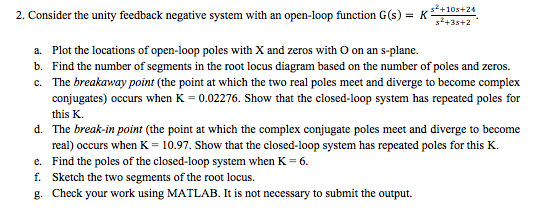2. Consider the unity feedback negative system with an open-loop function G(S)-KS. a. Plot the locations of open-loop poles with X and zeros with O on an s-plane. b. Find the number of segments in the root locus diagram based on the number of poles and zeros. c. The breakaway point (the point at which the two real poles meet and diverge to become complex conjugates) occurs when K = 0.02276. Show that the closed-loop system has repeated poles for...

• ###  Sketch the root locus for the unity feedback system whose open loop transfer function is K G(s) Draw the root locus...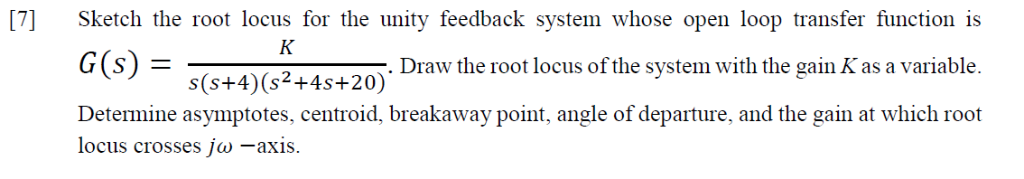Sketch the root locus for the unity feedback system whose open loop transfer function is K G(s) Draw the root locus of the system with the gain K as a variable s(s+4) (s2+4s+20)' Determine asymptotes, centroid,, breakaway point, angle of departure, and the gain at which root locus crosses jw -axis.  Sketch the root locus for the unity feedback system whose open loop transfer function is K G(s) Draw the root locus of the system with the gain...

• ### Problem 5. (20pts) The open-loop transfer function of a unity feedback system G(8) -- +2) a)...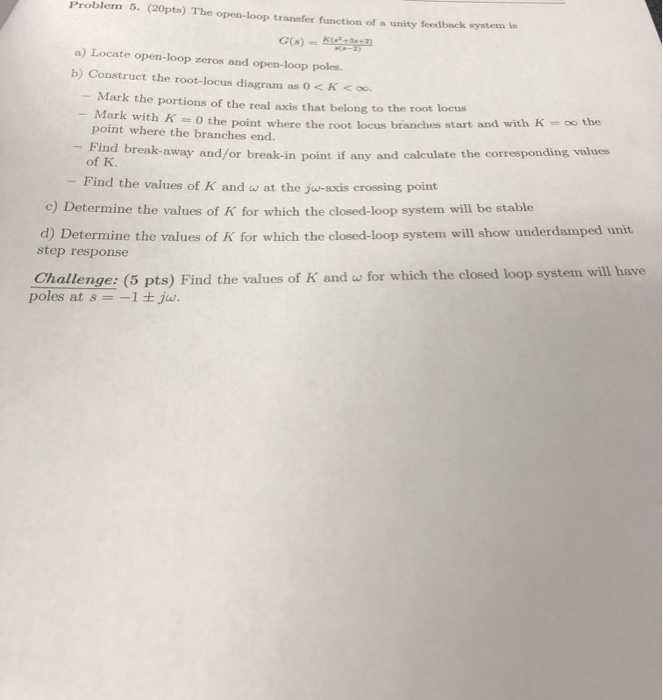Problem 5. (20pts) The open-loop transfer function of a unity feedback system G(8) -- +2) a) Locate open-loop zeros and open-loop poles. b) Construct the root-locus diagram as 0 <K <oo. Mark the portions of the real axis that belong to the root locus - Mark with K =0 the point where the root locus bra O the point where the root locus branches start and with K = oo the point where the branches end. - Find break-away and/or...

• ### 7. Consider a unity feedback control system with open-loop transfer function G(s) = k 5 s...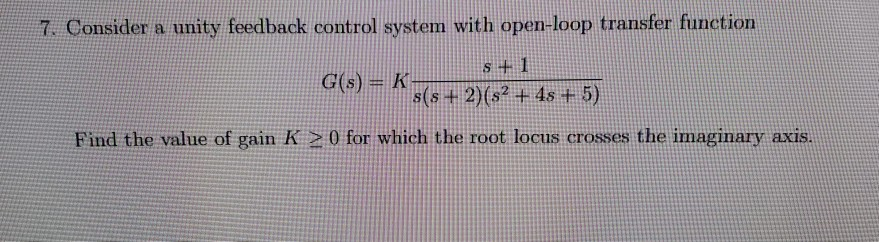7. Consider a unity feedback control system with open-loop transfer function G(s) = k 5 s + 2)(52 + 4s + 5) Find the value of gain K > 0 for which the root locus crosses the imaginary axis.

• ### Sketch the root locus plot of a unity feedback system with an open loop transfer function...

Sketch the root locus plot of a unity feedback system with an open loop transfer function G(s) = K / s (s+2) (s+4) Determine the value of K so that the dominant pair of complex poles of the system has a damping ratio of 0.5.

• ### 4. The open loop transfer function of a unity feedback control system is s 5 Showing...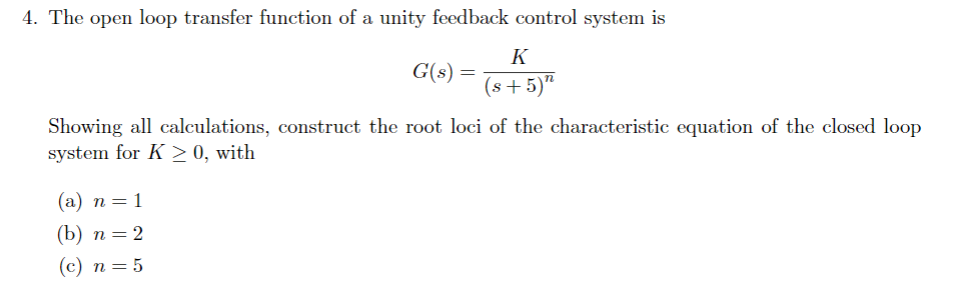4. The open loop transfer function of a unity feedback control system is s 5 Showing all calculations, construct the root loci of the characteristic equation of the closed loop system for K20, with (a) n-1 (c) n=5 n-

• ### Sketch the root locus for the unity feedback system shown in Figure P8.3 for the following...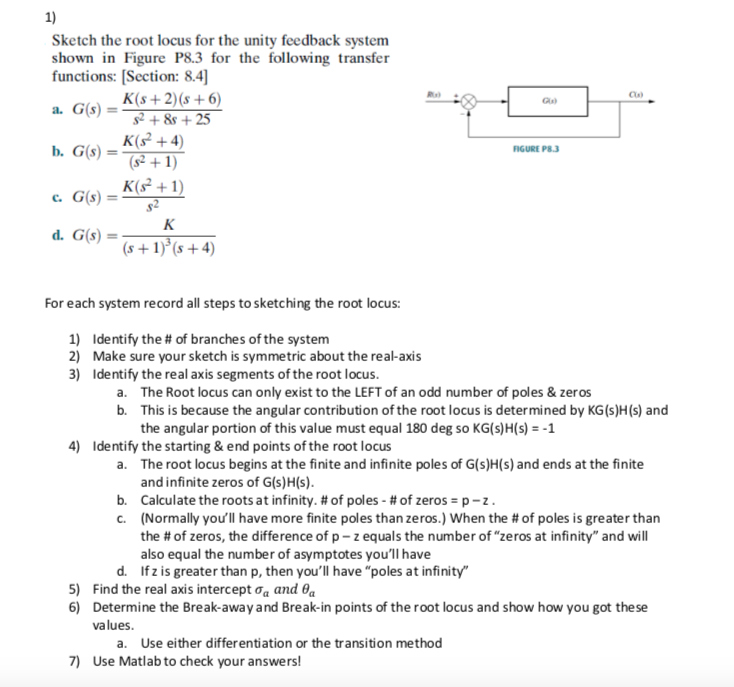Sketch the root locus for the unity feedback system shown in Figure P8.3 for the following transfer functions: (Section: 8.4] K(s + 2)(8 + 6) a. G(s) = 52 + 8 + 25 K( +4) b. G(S) = FIGURE PR3 152 +1) C G(s) - K(s+1) K (n1)(x + 4) For each system record all steps to sketching the root locus: 1) Identify the # of branches of the system 2) Make sure your sketch is symmetric about the real-axis...